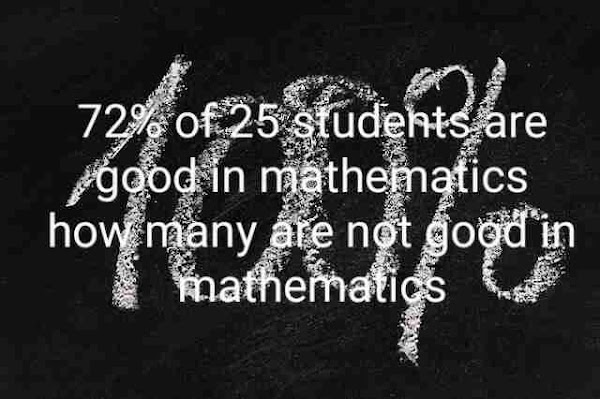## 72% of 25 students are good in mathematics how many not?

### 72% of 25 students are good in mathematics how many not?Monday, 4 October 2021

For some students, percentage calculation is quite tough. But for some that is as easy as nothing. So it will be necessary to know the percentage calculation. If you want to learn how to calculate percentage in more detail then click here.

Please note that normal percentage is quite different from aggregate percentage. That's why we have given both the links.

## What is percentage?

In simple words a percentage is nothing but just a number that is equally divided into 100 parts. Let's understand it in another way.

Percentage can be defined as the value or amount that indicates a definite part of 100 number.

Now, let's come to our main topic i.e,

### 72% of 25 students are good in mathematics how many are not good in mathematics.

Solution 1

So this question is ofcourse from percentage topic. Let's solve it.

As question said that there are total 25 number of students.

In 25 students. 72 percent of them are good in mathematics. Means they have strong math.

Now, the students who loves mathematics are given in percentage form. Let's change it in number form.

72 % of 25

72 / 100 × 25

72/4

18.

So there are total 18 students who are good in mathematics. And the total number of students in a class is 25. So if we subtract those students who loves mathematics in total number of students then the resultant will be of those students who doesn't like mathematics.

So, the required number (25 - 18) is equal to 7.

Hence, there are total 7 number of students who are not good mathematics.

Solution 2

As we know the 72% of 25 students like Mathematics. Find out how many do not like mathematics.

So, let's 'x' number of students who not like mathematics.

We have total number of students are 25.

So, the number of students who loves mathematics will be (25 - x).

But we have an estimate of 72 % students who like mathematics in total of 25. Here we have the estimate of students in percentage form.

Now, let's find how many students who loves mathematics in number form.

72 % of 25 = y

72 / 100 × 25 = y

After knowing 'x' and 'y' if we add both the number. We will get 25. i.e total number of students.

As we have solve in above solution that the number of students who loves mathematics are 18.

X + Y = 25

X + 18 = 25

X = 25 - 18

X = 7.

Hence, there are total 7 number of students who doesn't like mathematics.